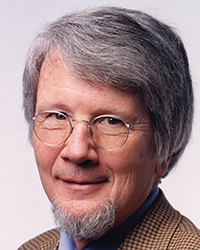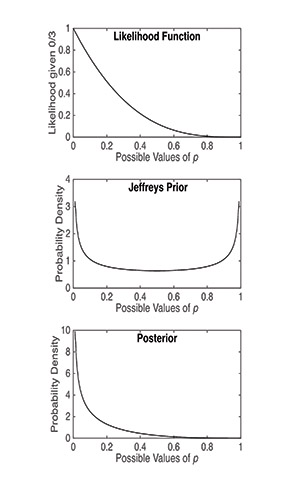# Bayes for Beginners 2: The Prior

In his inaugural Presidential Column, APS President C. Randy Gallistel introduced beginners to Bayesian statistical analysis. This month, he continues the introduction to Bayes with a lesson on using prior distributions to improve parameter estimates.In last month’s column, I focused on the distinction between likelihood and probability.

To review, probability attaches to the possible outcomes from a random process like coin flipping (known technically as a Bernoulli process). A probability distribution gives the probabilities for the different possible results given the parameters of the process. Suppose we are given a 50% chance of success (i.e., of flipping a head; p = .5) and told that there were 10 flips. Given these parameters, the probability of getting exactly 5 heads when flipping a coin 10 times is roughly .25.

Likelihood, by contrast, attaches to our parameter estimates and to our hypotheses. For example, given that we have observed 9 heads in 10 flips of a coin, the likelihood that the probability of flipping a head is 50% (i.e., that p = .5) is very low. The likelihood that p = .9 is greater by a factor of almost 40. The likelihood function tells us the relative likelihoods of the different possible values for p.

The likelihood function is only one of two components of a Bayesian calculation, however. The other is the prior, which is necessary for estimating parameters and for drawing statistical conclusions. Using prior distributions improves one’s parameter estimates and quantifies one’s hypotheses.

A prior distribution can and should take account of what one already knows. However, when one knows very little, one can use the Jeffreys priors, named after English mathematician Sir Harold Jeffreys, who helped revive the Bayesian view of probability. Jeffreys priors are some of the most interesting and useful prior distributions, and they derive from the mathematical implications of knowing absolutely nothing about the parameters one wants to estimate other than their possible ranges.

### Improving Parameter Estimates With a PriorA prior distribution assigns a probability to every possible value of each parameter to be estimated. Thus, when estimating the parameter of a Bernoulli process p, the prior is a distribution on the possible values of p. Suppose p is the probability that a subject has done X. Assume we initially have no idea how widespread this practice is. We ask the first three subjects whether they have done it. They all say, “No.” At this early stage, what proportion of the population should we estimate has done X? And how certain should we be about our estimate?

The data by themselves give p(X) = 0. That value specifies a distribution with no variance; it predicts that every subsequent subject also will not have done X. Our intuition suggests that it is unwise to take three people’s experiences as representative of all people’s experiences. The data at hand, however, do give us some information: We already know that p(X) ≠ 1 (because at least one subject has not done X), and it seems unlikely that p(X) > .9 (because none of our three subjects have done X).

Bayesian parameter estimation rationalizes and quantifies these intuitions by bringing a prior distribution into the calculation. The prior distribution represents uncertainty about the value of the parameters before we see data. Jeffreys realized that knowing nothing about a parameter other than its possible range (in this case, 0–1) often uniquely specifies a prior distribution for the estimation of that parameter.

The Jeffreys prior for the p parameter of a Bernoulli process is in the form called the beta distribution. The beta distribution itself has two parameters, denoted a and b. For the Jeffreys prior, these take the values a = b = .5. Following the common practice, I call these parameters hyperparameters to distinguish them from the parameter of the distribution that we are trying to estimate.

By adopting a Jeffreys prior, we can calculate a best estimate for p and quantify our current uncertainty about p at every stage of data gathering, from the stage where we have no data to the stage where we have an n in the millions. The Bayesian calculation requires multiplying the likelihood function by the prior distribution and normalizing the result in order to obtain the posterior distribution (i.e., a new distribution of probabilities for the different values of p, taking into account the data and the prior). This process sounds pretty intimidating.

When we use the Jeffreys prior, however, the posterior distribution takes the same form as the prior distribution; a beta distribution goes in as the prior and a beta distribution emerges as the posterior. (A prior distribution with this wonderful property is called a conjugate prior.) Thus, the only thing that the computation does is change the values of the parameters of a beta distribution. Moreover, the computation of the new values for these parameters is very simple: apost = aprior + ns and bpost = bprior + nf , where ns denotes the number of successes (in this case, subjects who have done X) and nf the number of failures (subjects who haven’t). The best estimate of p — the mean of the posterior distribution — is apost / (apost +  bpost). Statistical calculations never get easier than that.

Best of all, the resulting posterior distribution tells us how uncertain we should be about the true value of p. In traditional statistics, this is what the confidence interval is supposed to do. (It does it badly, but that’s another story.) Estimating a confidence interval for estimates of p when the sample is low is not straightforward, whereas the calculation of the posterior distribution using a conjugate prior is, as already explained, simplicity itself.

Figure 1 plots the likelihood function, the Jeffreys prior, and the posterior distribution for the case where we have three negatives and no positives. Notice how well Bayesian statistics can capture what our intuition tells us we can learn from this small sample.Gallistel correctly notes that probabilistic inferences based on new data should take into account what one already knows. The mathematical foundations and practical consequences of this were most thoroughly established by the statistical physicist Edwin T Jaynes (2003), who built on both Bayes and Jeffreys but went much further. To understand probabilistic inference and its neuronal basis it is crucial to see that there are two very different and complementary ways in which knowledge can be taken into account. The most obvious and best known way is via the prior probability of an inference calculated independently of the new data, i.e. via what is known as the prior. The other way is at least as important, i.e. via the likelihood. In contrast to calculation of the prior, calculating this depends upon knowing the new data, and it can be sensitive to knowledge of contextual information that is neither necessary nor sufficient for the calculation of posteriors, but which, if available, can have large effects on the calculation of likelihoods. Consider the possible interpretations of an ambiguous input. Some of those interpretations may be highly unlikely except in certain contexts. Thus, whereas priors may specify the probability of each interpretation averaged over all contexts, likelihoods can be used to greatly increase the probability of selecting posteriors that, though unlikely overall, are highly likely in the current context. It may well be that the role of knowledge in determining likelihoods is at last as fundamental as its role in determining priors.Hello Randy,
Much appreciation for these two columns! I was wondering if you might yourself, or ask someone, to do a third in this great series. I would love to see a data set or study discussed in terms of how data analysis would be different done by class null hypothesis methods vs a Bayesian analysis. I can almost understand the various examples offered in these two columns, but I think I (and perhaps others) would greatly benefit from a side by side contrast if that is possible?
Thank you!
JuliaThe plot that shows the Jeffreys prior confuses me because I thought that probability density functions can only max out at 1.Responding to Eric Garr: Probabilities cannot be greater than 1, but probability densities can have any value from 0 to +infinity. A probability density applies to a probability distribution with continuous support, e.g. durations or rates. The value of a probability density at any point (any support value) is the derivative of the cumulative probability distribution at that point. In other words, it is the rate at which probability is increasing at that point. If all of the probability is concentrated at a single point, as in a null hypothesis that predicts p=.5, then the rate of increase in probability at that point is infinite

This site uses Akismet to reduce spam. Learn how your comment data is processed.